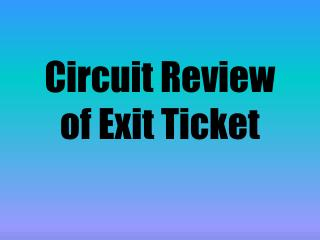DownloadDownload PresentationCircuit Review of Exit Ticket

# Circuit Review of Exit Ticket

Télécharger la présentation## Circuit Review of Exit Ticket

- - - - - - - - - - - - - - - - - - - - - - - - - - - E N D - - - - - - - - - - - - - - - - - - - - - - - - - - -
##### Presentation Transcript

1. Circuit Review of Exit Ticket

2. The correct answer is d • We know this is a parallel circuit because there is more than one route for the electrons (current) to take

3. The correct answer is a • The reason the answer is a is because it is a parallel circuit, therefore a switch at 2 would only turn off the first light bulb and a switch at 3 or 4 would only turn off the second light bulb

4. The correct answer is b • This is the correct answer because we need to complete the series circuit reconnecting the light bulb to the battery

5. The correct answer is d • All circuits must contain a wire, a load, and a source of emf

6. The correct answer is a • In a series circuit, if one load is broken/disconnected then all loads go out

7. The correct answer is d • We learned that batteries are powered by chemical reactions, which in turn power the electrical energy, which is used to shine the light

8. Circuit A Circuit B

9. SERIES CIRCUITS To solve for total current: It = I1 = I2 = I3 =… To solve for total voltage: Vt = V1 + V2 + V3 +… To solve for equivalent resistance: Req = R1 + R2 + R3 +… PARALLEL CIRCUITS To solve for total current: It = I1 + I2 + I3 + … To solve for total voltage: Vt = V1 = V2 = V3 = … To solve for equivalent resistance: 1/Req= 1/R1 + 1/R2 + 1/R3 + …